## Viscosity Unit:

Viscosity Unit is the property of a fluid which determines the amount of resistance to a shearing stress. A real fluid has no viscosity but it is non­existent.

Viscosity can also be defined as the property of a fluid due to which it offers resistance to the movement of one layer of fluid over another adjacent layer.

Viscosity increases with increase in temperature in case of gases whereas it decreases in case of liquid.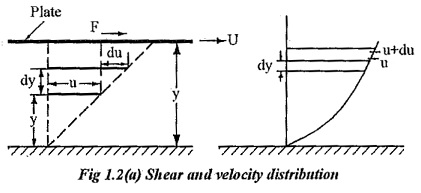Consider, a plate is placed at a distance of ‘Y’ from the fixed surface. The space in between is filled with a fluid. The plate moves with a velocity U by a force F as shown in fig 1.2. A fluid layer at a distance of y from surface moves with a velocity of `u’ and a layer at a distance of dy from y moves with a velocity of u+du.

According to Newton’s law of viscosity the shear force, F acting between two layers of fluid is proportional to difference in their velocities du and area A of the plate and inversely proportional to the distance dy between them..

Therefore,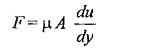Shear stress,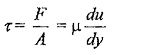Where,

μ (Mu) is the constant of proportionality or co-efficient of dynamic viscosity or Viscosity Unit. Its unit can be derived as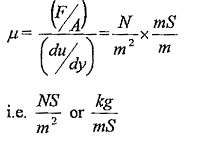du/dy – is the rate of shear deformation or rate of shear strain.

#### Dynamic Viscosity (μ):

As explained earlier, the dynamic viscosity (μ) is defined as the shear stress required causing unit rate of shear deformation.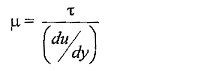#### Units of viscosity:

In metric system,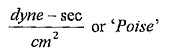1Poise = 1gm/cm-sec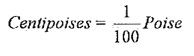In SI units,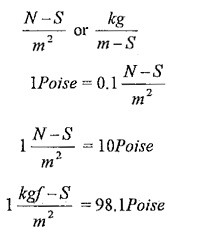#### Kinematic Viscosity (v):

The kinematic viscosity ( v) viscosity is defined as the ratio of dynamic viscosity to mass density.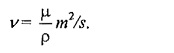The name kinematic viscosity has been given to the ratio (µ/p) because kinematics is defined as the study of motion without regard to the cause of motion and it concerned with length and time only. Likewise kinematic viscosity also involves the magnitudes of length and rime only.

#### Units:

In SI unit, m2/sec.

It is often measured in ‘stokes’.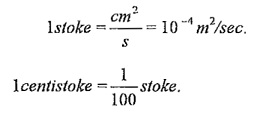In case of liquids, kinematic viscosity decreases with increase in temperature. In case of gases, it increases with increase in temperature.

Relative or Specific viscosity is the ratio of dynamic viscosity of any fluid to the dynamic viscosity of water at 20°C.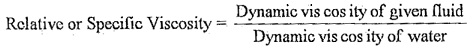Since, water has a viscosity of 1, it is taken as standard substance for relative viscosity.

#### Newton’s law of viscosity:

It states that the shear stress τ on fluid element, layer is directly proportional to the rate of shear strain. The constant of proportionality is called coefficient of viscosity.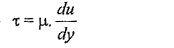The fluids which follow this law is known as Newtonian, fluids otherwise, it is known as Non-Newtonian fluids.

#### Effects of temperature on viscosity:

Variation of Viscosity Unit with respect o temperature for some common fluids are given in fig 1.2(b).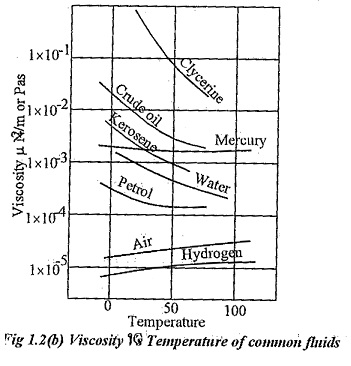In case of liquids, when the temperature increases the distance between molecules increases and the cohesive force decreases. So, viscosity of liquids decreases when the temperature increases.

In case of gases, the contribution to viscosity is more due to momentum transfer. When temperature increases, more molecules cross over with higher momentum differences. Hence, viscosity of gases increases with temperature.

Scroll to Top